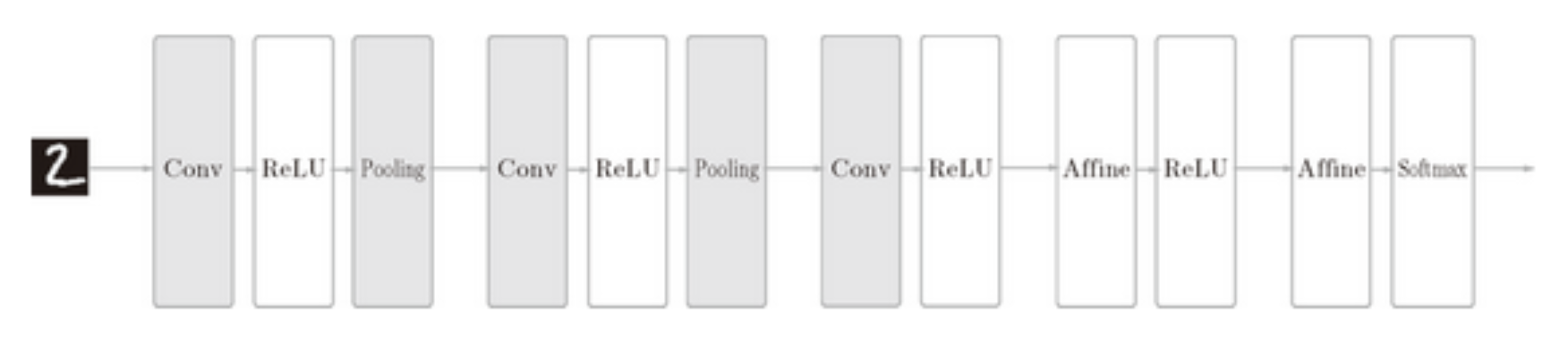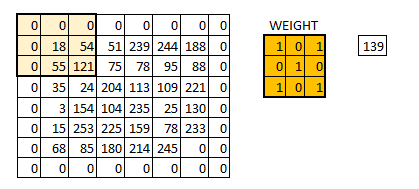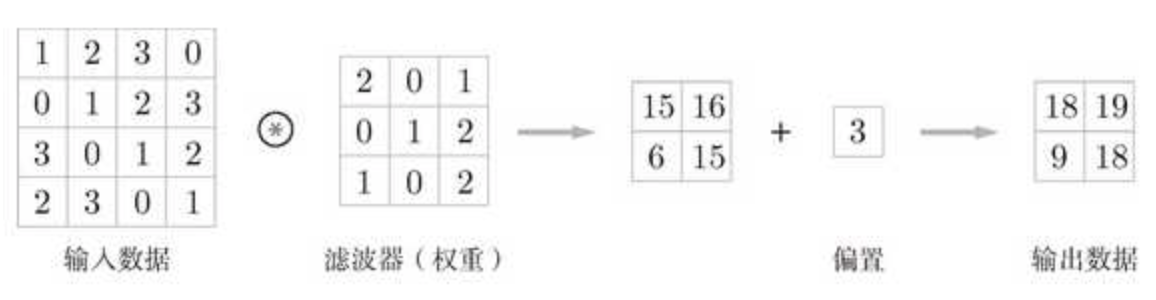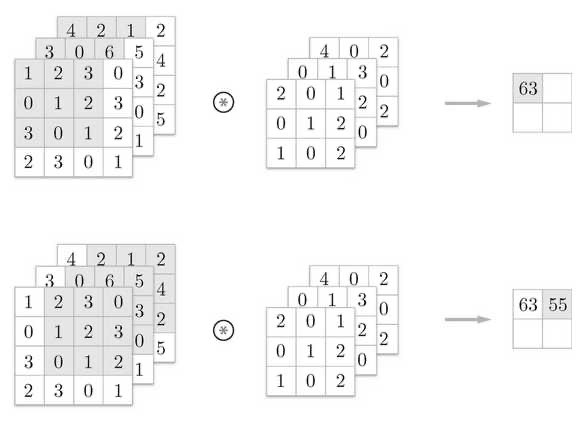# 从零开始的深度学习 Ch5

## Introduction to Convolutional Neural Network

Posted by R1NG on January 10, 2021 Viewed Times

# 卷积神经网络## 1. 卷积层和池化层Max 池化是获取最大值的运算, 在上图所示的运算中, 我们选定的目标区域大小是 $2*2$. 此外, 我们还将步幅设为了 $2$. 一般而言, 我们将池化窗口大小和步幅设为相同的值.

1. 和卷积层不同, 池化只是从目标区域中取最值, 池化层没有需要学习的参数.
2. 池化运算的计算是按照通道而独立进行的, 因此池化运算并不会改变输出数据和输入数据的通道数.
3. 一般而言, 在输入数据发生微小偏差时, 池化仍然会返回相同的结果. 这一特性也被称为池化运算的 健壮性.

## 2. 卷积层与池化层的实现

CNN 中, 各层间传递的数据维度为 $4$: 数据数量, 数据高, 数据长, 数据通道数.

1
2
3
4
x = np.random.rand(10, 1, 28, 28)

//the 1st data: x
//the 1st data's 1st channel data: x


1
2
3
4
5
6
7
8
9
10
11
12
13
14
15
16
17
18
19
20
21
22
23
24
25
26
27
28
29
30
def im2col(input_data, filter_h, filter_w, stride=1, pad=0):
"""

Parameters
----------
input_data : 由(数据量, 通道, 高, 长)的4维数组构成的输入数据
filter_h : 滤波器的高
filter_w : 滤波器的长
stride : 步幅

Returns
-------
col : 2维数组
"""
N, C, H, W = input_data.shape
out_h = (H + 2*pad - filter_h)//stride + 1
out_w = (W + 2*pad - filter_w)//stride + 1

col = np.zeros((N, C, filter_h, filter_w, out_h, out_w))

for y in range(filter_h):
y_max = y + stride*out_h
for x in range(filter_w):
x_max = x + stride*out_w
col[:, :, y, x, :, :] = img[:, :, y:y_max:stride, x:x_max:stride]

col = col.transpose(0, 4, 5, 1, 2, 3).reshape(N*out_h*out_w, -1)
return col


1
2
3
4
5
6
7
8
9
10
11
12
13
14
15
16
17
18
19
20
class Convolution:
def __init__(self, W, b, stride=1, pad=0):
self.W = W
self.b = b
self.stride = stride

def forward(self, x):
FN, C, FH. FW = self.W.shape
N, C, H, w = x.shape
out_h = int(1 + (H + 2*self.pad-FH) / self.stride)
out_w = int(1 + (w + 2*self.pad-FW) / self.stride)

col = im2col(x, FH, FW, self.stride, self.pad)
col_W = self.W.reshape(FN, -1).T
out = np.dot(col, col_W) + self.b

out = out.reshape(N, out_h, out_w, -1).transpose(0, 3, 1, 2)

return out


1
2
3
4
5
6
7
8
9
10
11
12
13
14
15
16
17
18
19
class Pooling:
def __init__(self, pool_h, pool_w, stride=1, pad=0):
self.pool_h = pool_h
self.pool_w = pool_w
self.stride = stride

def forward(self, x):
N, C, H, W = x.shape
out_h = int(1 + (H - self.pool_h) / self.stride)
out_w = int(1 + (W - self.pool_w) / self.stride)

col = im2col(x, self.pool_h, self.pool_w, self.stride, self.pad)
col = col.reshape(-1, self.pool_h*self.pool_w)

out = np.max(col, axis=1)
out = out.reshape(N, out_h, out_w, C).transpose(0, 3, 1, 2)

return out